# Excel Tutorial : How to count and add cells by color

#### EXCEL course learning outcomes

This tutorial explains how to count and add cells by font color or cell color in Excel. These solutions work for both manually colored cells and with conditional formatting.

If you have a spreadsheet in which you use various filler colors and fonts to differentiate different types of cells or values, you may want to know how many cells are highlighted with a certain color. If the contents of your cells are in number format, you can also automatically calculate the sum of the cells with the same fill color, for example the sum of all red cells.

Wouldn’t be great if there was a function that could count the colored cells in Excel? Unfortunately, there is no built-in function for this purpose. But you can easily do it.

The NB function in Excel counts cells containing numbers in the cell range as argument. You cannot use this function to count colored or highlighted cells. However, you can follow some workaround solutions in order to count colored cells in Excel. Follow the rest of the tutorial for more details.

## Table of contents

How to Count the colored cells in Excel

Method 1 : Use of the NB.SI and READ.CELL functions

Method 2 : count the colored cells using the filtering and SUB-TOTAL function

Method 3: count colors using VBA (by creating a custom function)

Sum of cells by font color with VBA code

Sum of cells per fill color with VBA code

Count cells by font color with VBA code :

In the first section, we will see how to count colored cells in Excel with three different methods:

## How to Count the colored cells in Excel

In an Excel spreadsheet, you can count highlighted cells by using one of the following workaround solutions:

• Use of the NB and READ.CELL functions
• Application of SUB.TOTAL and data filtering
• Use the VBA

### Method 1 : Use of the NB.SI and READ.CELL functions

In this method, we are going to create a range named with the READ.CELL function, get the code color of each cell, then count or add easily the code of the wished color in Excel.

READ.CELL is an old Macro4 function and does not work with normal functions. However, it still works with named ranges. To count the colored cells with READ.CELL, you must extract the color codes with GET.CELL and count them to know the number of highlighted cells with the same color. To count the cells using READ.CELL and NB.SI, follow the steps below:

1. Create a named range :
• Go to « Formulas » tab and click on « Define a name » in the « defined names » group.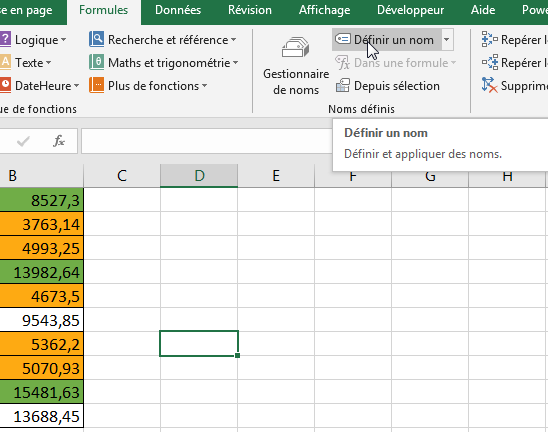• In the « New name » dialog box, enter :
• Name : Codecolor
• Area : Workbook
• Refers to : `=READ.CELL(38; \$B2)`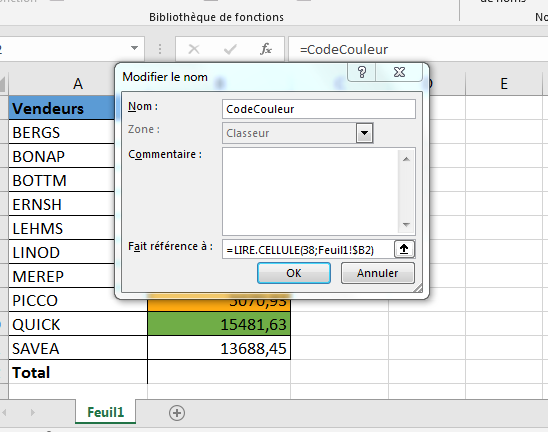• Click on « OK » to close the « New name » dialog box.

In the above formula, we used B2 as the second argument. You must use the column reference in which you have the cells with the background color.

1. Obtain color code for each cell :
• Enter the formula  `=ColorCode `in the cell  C2 :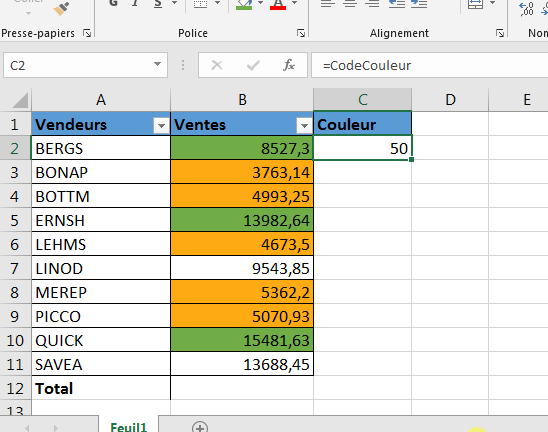• Drag the copy handle up to C11 with your mouse :This formula returns 0 if there is no fill color in a cell and returns a specific number if there is one. This number is specific to a color, so all cells with the same background color receive the same number.

1. Count the colored cells using the calculated color code:

If you follow the above process, you would have a column with numbers corresponding to the fill color of each cell. To count cells of a specific color follow the steps below:

• Somewhere under the dataset, apply the same fill color that you want to count to a given cell. Make sure you do this in the same column you used to create the named range. For example, we used column B, so we will only use the cells in column B.
• In the adjacent cell (C13 in this example), use the following formula: `=NB.SI(\$C\$2:\$C\$11; ColorCode) `: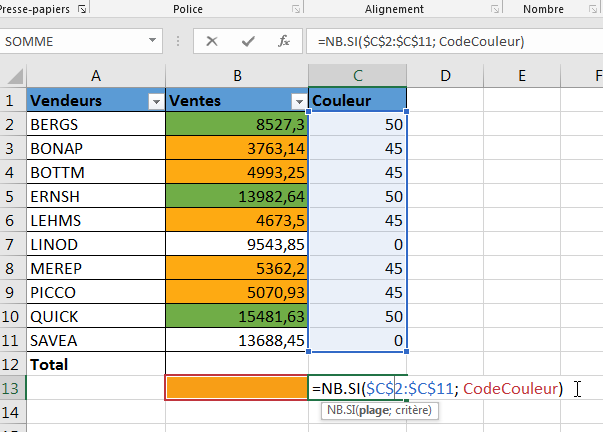This formula will count the orange colored cells to find the number of sellers with sales below 7000 DH and return the number 5.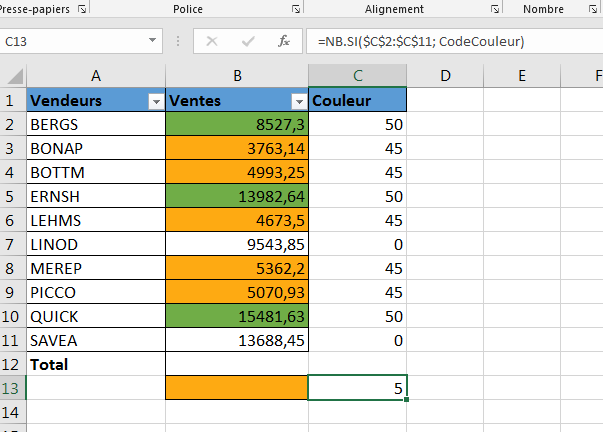The NB.SI function uses the range named « ColorCode» that we created, as a criteria. The range named in the formula refers to the adjacent cell on the left (in column B) and returns the color code of that cell. Therefore, this color code number is the criterion.

The NB.SI function uses the « \$C\$2:\$C\$11 » range that contains the numbers of the color code of all the cells and returns the number bases on the code sent by ColorCode in the criterion.

Now we are going to move to the next method where you can count the colored cells by using data filtering and the SUB.TOTAL function.

### Method 2 : count the colored cells using the filtering and SUB-TOTAL function

You can count cells with a certain fill color in Excel by sub-totaling the visible cells and applying a color-based filter.
In this example, we have the sales records of ten sellers for the month of September. Lines containing sellers with sales below 7,000 DH are highlighted in orange, the other cells with sellers with bonuses are highlighted in green.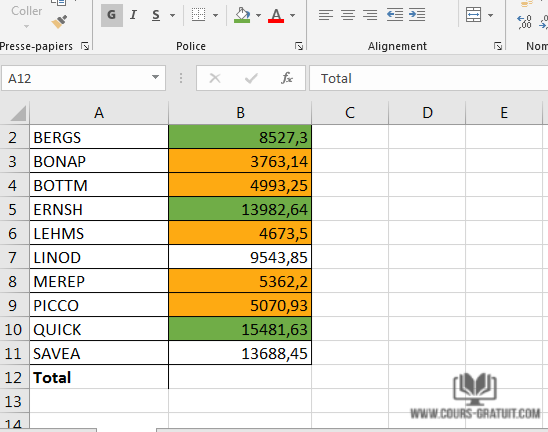To count the colored cells in Excel, you have to follow the two steps bellow:

• Filter the colored cells ;
• Use SUB.TOTAL function to count visible colored cells (after filtering) .

Two background colors are used in this dataset (green and orange). To calculate the number of vendors highlighted in orange, follow these steps:

1. Select the cell  B12.
2. Enter the formula `=SOUS.TOTAL(102; B2:B11)`.  The first argument 102 counts visible cells within the specified range :1. Select A1 :B11 cells by clicking on A1 cell and dragging it to B11 with your mouse .
2. Access « Data » tab .
3. In the « Sort and filter » group click on « Filter ». This will apply a filter to all headings in your table :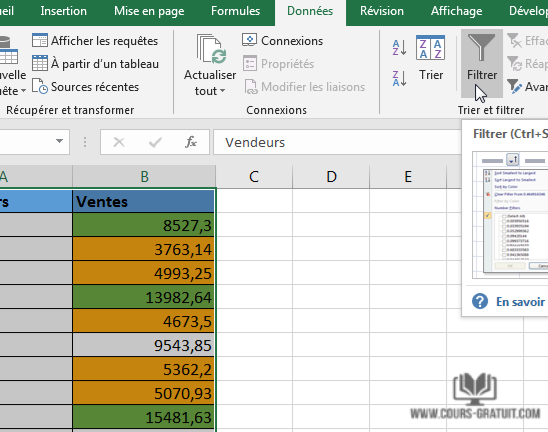1. Click the B1 filter drop-down list .
2. Click on the « Filter by color » option .In the above data set, since two colors are used to highlight the cells, the filter displays two colors to filter those cells. Select the orange color to discover the sellers highlighted in orange color.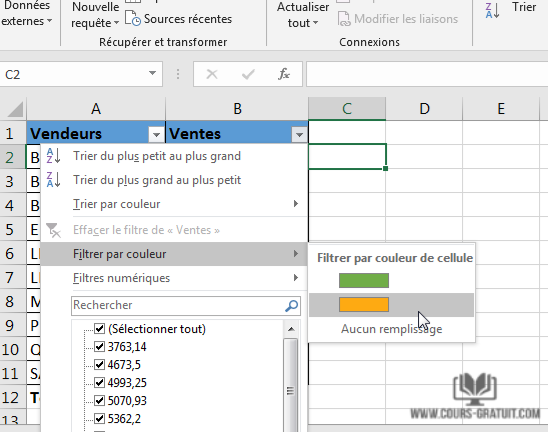As soon as you filter the cells, you will notice that the value of the SUB.TOTAL function changes and returns only the number of visible cells after filtering. The SUB.TOTAL function uses the number 102 as a first argument, which is used to count visible cells (hidden rows are not counted) in the specified range .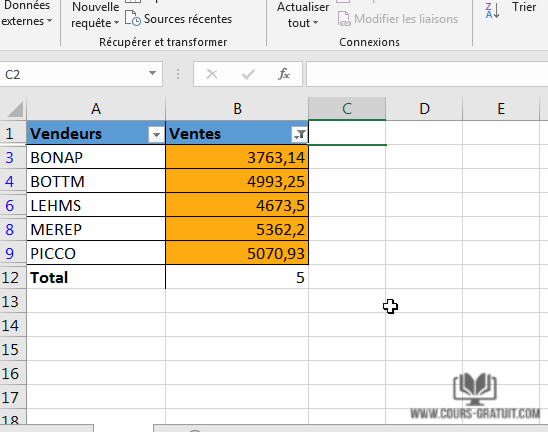If the data is not filtered, it returns 10, otherwise , it returns only the number of visible cells .

Finally we will see the last method that counts colors using VBA .

### Method 3: count colors using VBA (by creating a custom function)

Another workaround method is to create a custom function with VBA to count cells with a color in Excel. To do this, you need to create a custom function using VBA that works as a NB.SI function and returns the number of cells for a certain color.

You will follow the syntax: =CountColor(ColourMap; Color) and use it like other regular functions. Here, Color is the color for which you want to count the cells. PlageColor is the range in which you want to count the cells with the specified background color.

To create this custom function follow the steps below:

1. Open the workbook containing your data, press Alt + F11 to open the VBA editor. You can also open it by right-clicking on the spreadsheet tab and selecting  « View code» :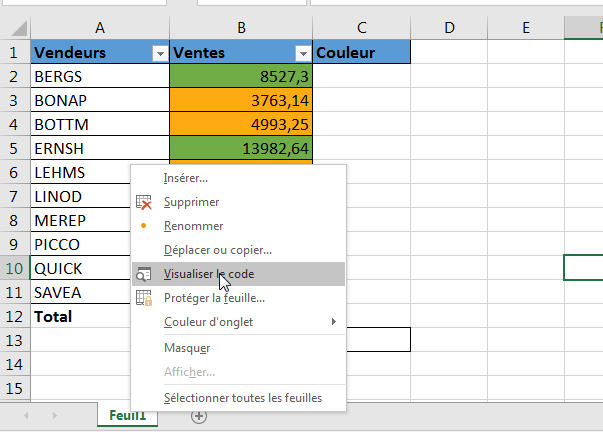1. In the left pane, under the name of the workbook you are working in, right-click one of the worksheets and select« Insert »   then choose « Module » . This would insert a new module:1. Copy and paste the code into the module code window.

`Function CompterCouleur(PlageCouleur As Range, Couleur As Range)Dim CodeCouleur As IntegerDim NbrCouleur As IntegerCodeCouleur = Couleur.Interior.ColorIndexSet CCell = PlageCouleurFor Each CCell In PlageCouleur  If CCell.Interior.ColorIndex = CodeCouleur Then    NbrCouleur = NbrCouleur + 1  End IfNext CCellCompterCouleur = NbrCouleurEnd Function`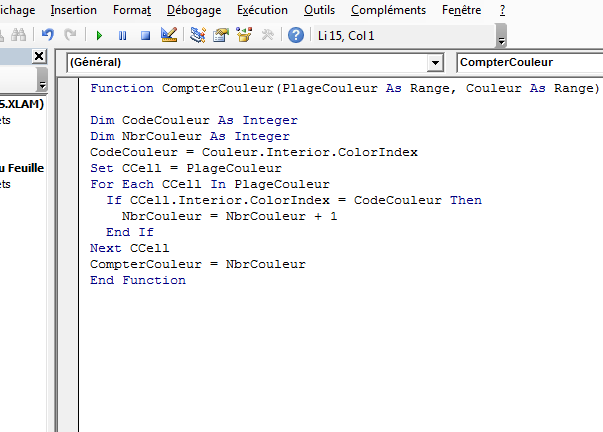In this code, we defined a function with two arguments ColorRange and Color . We will record the fill color value of cell B2 in ColorCode. Then we’ll run a FOR loop where, if the cell’s background color matches the color of ColorCode, you increment ColorNbr. This function returns the value of ColorNbr, which is the number of cells with the same background color.

In this code, we defined a function with two arguments ColorRange and Color .

1. Close the VB Editor. That’s it! You now have a custom function in the spreadsheet called CompterCouleur.
2. Now we will use this formula. Use the background color you want to count in a cell (Here, B13) and use the following formula in the adjacent cell (C13):

`=CompterCouleur(B2:B11;B13)`

Here B13 is the cell with the background color (orange) that you want to count. B2:B11 is the range of cells in which you want to count the color.

This will return the number of sellers with sales below 7000 DH in September, or 5.If, after applying the VBA code mentioned above, you need to manually color a few additional cells, the sum and number of the colored cells will not be automatically recalculated to reflect the changes. Don’t be mad at us, it’s not a code bug.

In fact, this is the normal behavior of all Excel macros, VBA scripts and user-defined functions. The idea is that all these functions are called with a change in a spreadsheet data only and Excel does not perceive the change in font color or cell color as a change in the data. So, after applying a fill color to cells manually, simply hover over any cell and press F2 and “Enter”, the sum and number will be updated.

Note:  Since there is a code in the workbook, save it as .xls or .xlsm file .

## Sum of cells by font color with VBA code

In this section you will learn how to add cells per font color in a given cell range in an Excel spreadsheet. We will also write a user-defined function to get the result. Simply follow the steps:

1. Open the VBA editor by clicking on Alt+F11 shortcut, or by clicking on the « Visual Basic » command under the « Developer » tab .
2. Right-click a spreadsheet in the workbook and click « Insert» and the « Module» to create a new module :1. Paste the VBA code below into the  window code  :

`Public Function SommePrCoulDeFond(Plage As Range, Couleur As Range)     Somme = 0     For Each rCell In Plage         If rCell.Font.Color = Couleur.Font.Color Then             Somme = Somme + rCell.Value         End If     Next     SommePrCoulDeFond = SommeEnd Function`

`=SommePrCoulDeFond(B2:B11; B13)`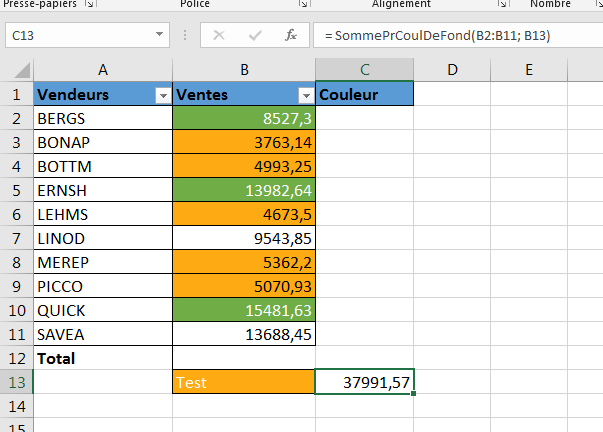## Sum of cells per fill color with VBA code

You can also create a VBA function to get the result of the sum of cells per cell fill color in Excel. Simply follow the steps below:

1. Open the VBA editor using the Alt+F11 shortcut, or by clicking on « Visual Basic » command under the « Developer» tab.
1. Right-click a spreadsheet in the workbook and click on « Insert » then on « Module » to create a new module :1. Copy and paste the VBA code below into the code window.
2. Then click the « Save » button and close the VBA editor.

`Public Function SommeParCouleur(plage As Range, Couleur As Range)      Somme = 0      For Each rCell In plage          If rCell.Interior.Color = Couleur.Interior.Color Then              Somme = Somme + rCell.Value          End If      Next      SommeParCouleur = Somme     End Function``=SommeParCouleur(B2:B11;B13)`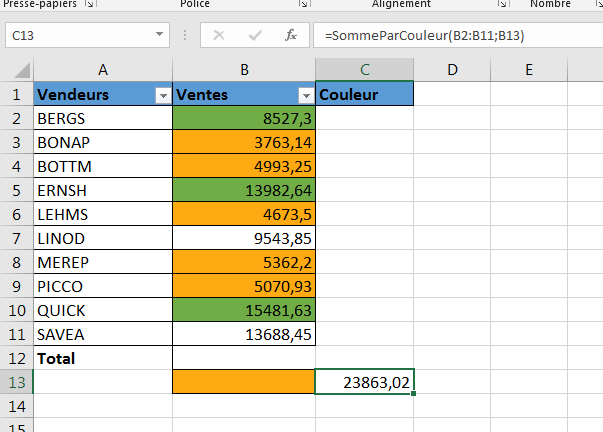## Count cells by font color with VBA code :

In this example we will count cells with a white font color in cell range B2:B11 . We will use the function below to get the result.

`Public Function CompterParCouleurFond(Plage As Range, Couleur As Range)     Application.Volatile     For Each Cellule In Plage         If Cellule.Font.Color = Couleur.Font.Color Then             CompterParCouleurFond = CompterParCouleurFond + 1         End If     NextEnd Function`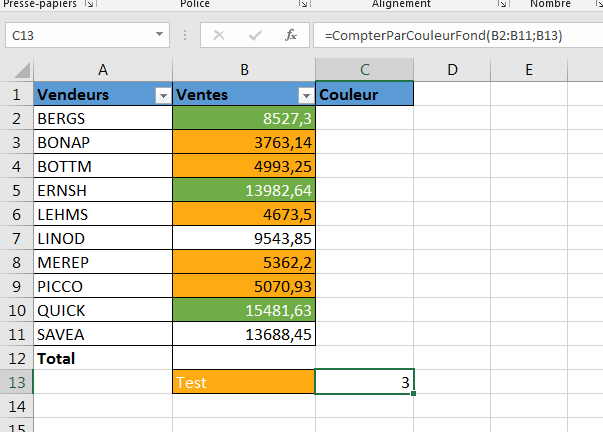## Conclusion:

Now that you have finished this tutorial, you know how to count the colored cells in Excel using different methods. So you can work at ease with your Excel file without any constraints.